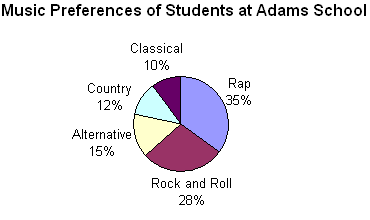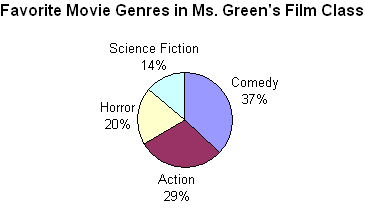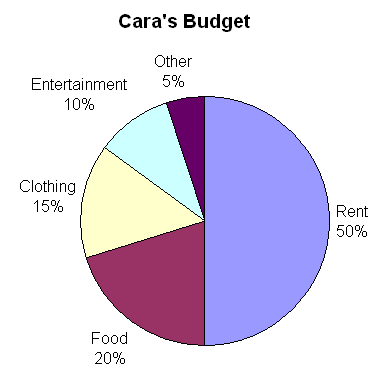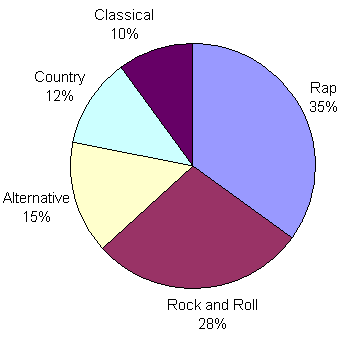# Constructing Circle Graphs

In the last lesson, we learned that a circle graph shows how the parts of something relate to the whole. A circle graph is divided into sectors, where each sector represents a particular category. Circle graphs are popular because they provide a visual presentation of the whole and its parts. However, they are best used for displaying data when there are no more than 5 or 6 sectors and when the values of each section are different.

Below are the circle graphs from each example in the last lesson. Do you notice a pattern in the way these 4 circle graphs are drawn?You will notice that in each circle graph above, the sectors are ordered by size: The sectors are drawn from largest to smallest in a clockwise direction. This information will help us as we learn how to draw a circle graph.

Example 1: The table below shows how Cara spends each part of her earnings. Construct a circle graph to visually display this data.

 Cara's Budget Expense Percent Rent 50% Food 20% Clothing 15% Entertainment 10% Other 5%

Step 1: Find the whole.

What is the total value for the items on your graph? This is usually 100% or 1 whole.

Adding all of the expenses together, we get 50% + 20% + 15% + 10% + 5% = 100%. The total value for all items is 100%.

Step 2: Find the parts.

Each item to be graphed represents a part of the whole. To complete the circle graph, you must find exactly what fraction or percent each item represents. The easiest way to do this is to take the quotient of the part and the whole and then convert the result to a percent.

The percent that each item represents was given in the table above.

Step 3: Find the degrees for each part.

Every circle is made up of 360 degrees. To find the angle measure for each item, use this formula:

angle measure for an item = percent the item represents x 360 degrees

 Cara's Budget Expense Percent Decimal Degrees Rent 50% 0.50 0.50 x 360° = 180° Food 20% 0.20 0.20 x 360° = 72° Clothing 15% 0.15 0.15 x 350° = 54° Entertainment 10% 0.10 0.10 x 360° = 36° Other 5% 0.05 0.05 x 360° = 18° Total 100% 1.00 = 1 360°

Step 4a: Draw the parts.

Draw a circle and a radius. Use a protractor to draw each angle. Each new angle should be measured from the previously drawn line segment. Draw the angles from largest to smallest in a clockwise direction.

Step 4b: Label each part of your graph.

Step 5: Give the graph a title.Example 2: The table below shows the music preferences of 300 students at Adams School. Each student voted only once. Construct a circle graph to represent this data.

 Music Preferences of Students at Adams School Music Type Number of Students Rap 105 Rock and Roll 84 Alternative 45 Country 36 Classical 30

Step 1: Find the whole.

What is the total value for the items on your graph? This is usually 100% or 1 whole.

The total value for all items is 300 students (or 1 whole school).

Step 2: Find the parts.

Each item to be graphed represents a part of the whole. To complete the circle graph, you must find exactly what fraction or percent each item represents. The easiest way to do this is to take the quotient of the part and the whole and then convert the result to a percent.

We know from the last lesson that a circle graph is easier to read when a percent is used to label the data. We need to find the percent that each item represents.

 Music Preferences of Students at Adams School Music Type Number of Students Percent Rap 105 105 ÷ 300 x 100 = 35% Rock and Roll 84 84 ÷ 300 x 100 = 28% Alternative 45 45 ÷ 300 x 100 = 15% Country 36 36 ÷ 300 x 100 = 12% Classical 30 30 ÷ 300 x 100 = 10% Total 300 100%

Step 3: Find the degrees for each part.

Every circle is made up of 360 degrees. To find the angle measure for each item, use this formula:

angle measure for an item = percent the item represents x 360 degrees

 Music Preferences of Students at Adams School Music Type Number of Students Percent Decimal Degrees Rap 105 35% 0.35 0.35 x 360° = 126° Rock and Roll 84 28% 0.28 0.28 x 360° = 100.8° Alternative 45 15% 0.15 0.15 x 350° = 54° Country 36 12% 0.12 0.12 x 360° = 43.2° Classical 30 10% 0.10 0.10 x 360° = 36° Total 300 100% 1.00 = 1 360°

Step 4a: Draw the parts.

Draw a circle and a radius. Use a protractor to draw each angle. Each new angle should be measured from the previously drawn line segment. Draw the angles from largest to smallest in a clockwise direction.

Step 4b: Label each part of your graph.Step 5: Give the graph a title.Note that the area of each sector is in the same proportion to the whole circle as the number for each item in the table is to the total of all items.

Summary: There are 5 steps for constructing a circle graph. These steps are listed below.

 Procedure for Constructing a Circle Graph Step 1: Find the whole. Step 2: Find the parts. Step 3: Find the degrees for each part. Step 4: Draw and label each part. Step 5: Give the graph a title.

### Exercises

Directions: Use the procedure above to construct a circle graph for each table in the exercises below. You may print and use our circle graph paper (PDF).

Exercise 1: The revenue sources of the federal government were recorded as shown in the table below. Construct a circle graph to visually display this data.

 Revenue Sources for the Federal Government Source Percent Individual Income Tax 40 Social Security 33 Corporate Income Tax 17 Other 10